Learn To Invest
Stocks Special Reports LICs Credit Funds ETFs Tools SMSFs

# Standard Deviation and Sharpe Ratio

Morningstar.com.au  |  26 Jul 2022Text size|## Standard Deviation

Standard deviation is the statistical measurement of dispersion about an average, which depicts how widely a stock or portfolio’s returns varied over a certain period of time. Investors use the standard deviation of historical performance to try to predict the range of returns that is most likely for a given investment. When an investment has a high standard deviation, the predicted range of performance is wide, implying greater volatility.

If an investment’s returns follow a normal distribution, then approximately 68 percent of the time they will fall within one standard deviation of the mean return of the investment, and 95 percent of the time within two standard deviations. For example, for a portfolio with a mean annual return of 10 percent and a standard deviation of two percent, you would expect the return to be between eight and 12 percent about 68 percent of the time, and between six and 14 percent about 95 percent of the time.

In this context, the mean annual return is based on an arithmetic average (sum/n) of the monthly returns of the investment (an average, which is then annualized). This is slightly different than the total return of the investment, because the total return is a geometric average of the monthly returns, calculated as [(1+r1)(1+r2)…(1+rn)]-1.

Morningstar calculates standard deviation for stocks, open-end mutual funds, closed-end funds, exchange-traded funds, indexes, separate accounts, variable annuity underlying funds, and variable annuity sub-accounts. Morningstar uses the historical monthly total returns for the appropriate time period (one-, three-, five-, 10-, 15-, and 20-year) to calculate the monthly standard deviation. The monthly standard deviation is then annualized to put it into a more useful one-year context.

Morningstar uses the sample standard deviation method1. The monthly standard deviation is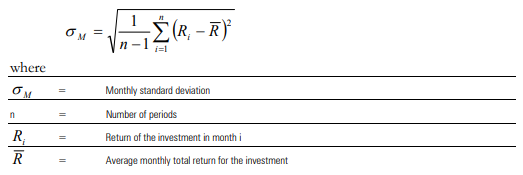R̄  is also called the arithmetic mean, and it is calculated by adding together all the monthly returns for the portfolio and dividing by the number of months.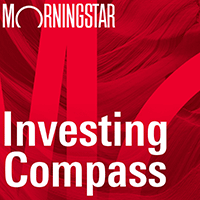Listen to Morningstar Australia's Investing Compass podcast
Take a deep dive into investing concepts, with practical explanations to help you invest confidently.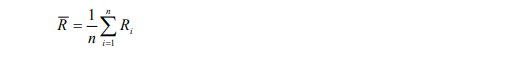Morningstar annualizes the monthly standard deviation to put the number in more useful one-year terms by multiplying it by the square root of 12.2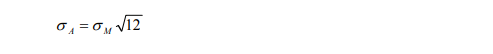Morningstar tools and websites most commonly display the annualized version of the three-year standard deviation.

## Sharpe Ratio

The Sharpe Ratio is a risk-adjusted measure developed by Nobel Laureate William Sharpe. It is calculated by using excess return and standard deviation to determine reward per unit of risk. The higher the Sharpe Ratio, the better the portfolio’s historical risk-adjusted performance. Morningstar calculates the Sharpe Ratio for portfolios for one, three, five, and 10 years. Morningstar does not calculate this statistic for individual stocks. The monthly Sharpe Ratio is as follows: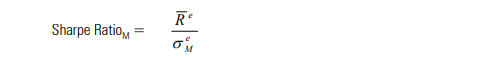The numerator, e , is the average monthly excess return: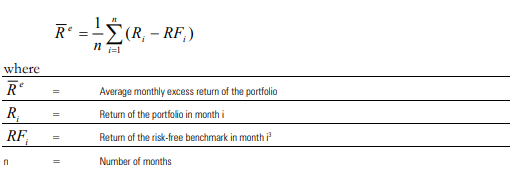The denominator, σeM, is a monthly measure of the standard deviation of excess returns. Because this measures the standard deviation of the spread between the portfolio and the risk-free rate, it is slightly different than the standard deviation of total returns displayed in most Morningstar products. The denominator is: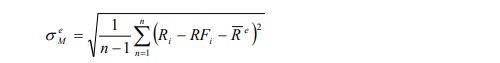The annualized Sharpe Ratio is the product of the monthly Sharpe Ratio and the square root of twelve. This is equivalent to multiplying the numerator by 12 (to produce an arithmetic annualized excess return) and the denominator by the square root of 12 (annualized standard deviation).4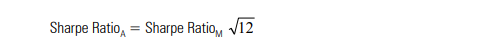1 This corresponds to the Microsoft Excel function “stdev.”

2 Prior to 2/28/2005, Morningstar calculated standard deviation with the population method (divide by n instead of n-1, “stdevp” in Microsoft Excel). Also, prior to this date, Morningstar annualized standard deviation with a method developed by James Tobin. σA=([(σM)2 + (1+avgR)2]12 – [(1+avgR)2]12)1/2

3 Morningstar chooses a risk-free benchmark based on the portfolio’s domicile, e.g. the 3-month Treasury bill for portfolios based in the United States.

Prior to 2/28/2005, Morningstar annualized the Sharpe Ratio with a method developed by James Tobin.

.